Site: Whipple, F. J. W.

## The Francis J. W. Whipple (1876-1943) Formulae for Associated Legendre Functions

### I have noticed a lot of people seem to like this site. So, I have made some attempt to improve the layout of the page. Please enjoy these transformations for yourself and may peace be with you during these difficult times. HSC 12/6/02

Another aspect of the symmetric nature of toroidal functions under index interchange is further demonstrated by using Whipple's formulae for associated Legendre functions (§3.3.1 in Erdélyi et al. 1953). We start with eqs. (8.2.7) and (8.2.8) in Abramowitz and Stegun (1965), namely,

These Whipple formulae have been presented previously in terms of a viewpoint aimed at spherical harmonics, now that we view the equations in terms of toroidal coordinates, whole new symmetries of Legendre functions arise.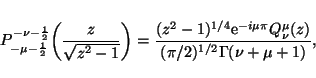(28)

and,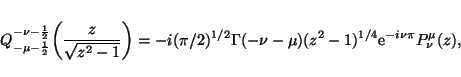(29)

which are valid only for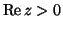(since the Legendre function of the second kind becomes discontinuous on the cut) and for all complex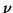and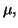except when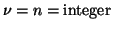since the Gamma function has poles along the negative real axis. By combining eqs. (28) and (29) with eqs. (20)--(23) along with the negative argument condition for Gamma functions [eq. (6.1.17) of Abramowitz & Stegun (1965)], namely,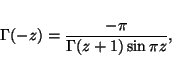(30)

we can write two general expressions which are valid for all complexand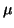. Here, for associated Legendre functions of the first kind,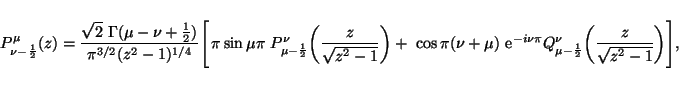(31)

which is equivalent to,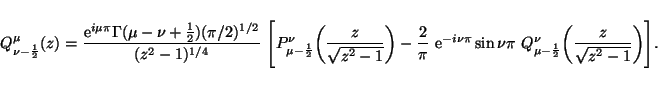(32)

## The Francis J. W. Whipple (1876-1943) Formulae for Toroidal Functions

Now, we substitute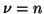and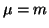(with m and n being positive integers) in (28) and (29) in order to derive the Whipple formulae for associated toroidal functions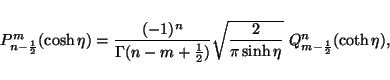(33)

which is equivalent to,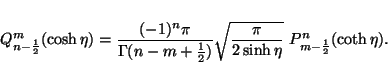(34)

These last two expressions allow us to express toroidal functions of a certain kind (first or second, respectively) with argument hyperbolic cosine, as a direct proportionality in terms of the toroidal function of the other kind (second or first, respectively) with argument hyperbolic cotangent. The Whipple formulae may also be expressed as follows: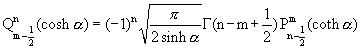,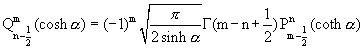,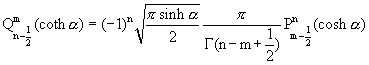, and,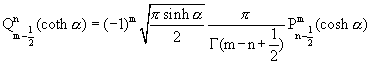.

These interesting formulae have the property that they can relate Legendre functions of the first and second kinds directly in terms of each other. The only hitch is that you need a different argument to relate them. The way it works it as such. The Legendre functions of the first kind generally are well-behaved near the origin and blow up at positive infinity. Consequently the Legendre functions of the second kind blow up at unity and exponentially converges towards zero for large values of the argument. The relevant domain for toroidal functions is from 1 to infinity. The standard hyperbolic argument for these functions are naturally chosen to be the hyperbolic cosine since it ranges from 1 to infinity. The Whipple formulae relate the Legendre functions with argument 1 to infinity, cosh, to a reversed range given by the hyperbolic cotangent function. the hyperbolic cotangent function ranges from infinity at unity to unity at infinity. At what point alpha does cosh alpha equal coth alpha? The point alpha is given by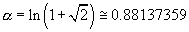.
Therefore, exp(alpha) and exp(-alpha) are respectively given by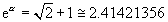,
and.
The value that cosh alpha and coth alpha obtain at alpha is given by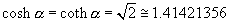.
The value that sech alpha and tanh alpha obtain at alpha is given by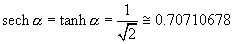.
Finally, sinh alpha and it's inverse csch alpha are given respectively by unity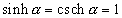.
We now see that the value at which the argument of the Legendre functions inversely maps the entire domain is given by cosh alpha = coth alpha ~ 1.4142356. By using the Whipple formulae for ring functions, we can inversely map the entire domain from 1 to infinity about this point cosh alpha, the square root of 2, and take full advantage of this new symmetry for Legendre functions. There being previously more definite and indefinite integrals tabulated for the Legendre function of the first kind than for the Legendre function of the second kind. In fact, this new transformation, when applied to toroidal functions yields distinct expressions which relate correspondingly the complete elliptic integrals of the first and second kind, which don't seem to be related to the linear and quadratic transformations of hypergeometric functions.